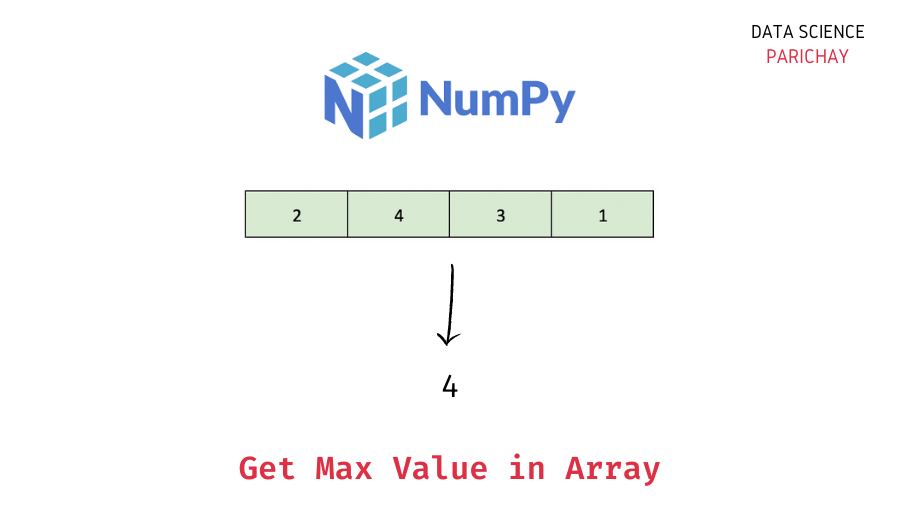# Numpy – Get Max Value in Array

The Numpy library in Python comes with a number of built-in functions to help get common descriptive statistics like max, min, mean, median, etc. from arrays. In this tutorial, we will look at how to get the max value in a Numpy array with the help of some examples.

## How to find the max value of a Numpy array?

You can use the Numpy `amax()` function to get the max value of a Numpy array. Pass the array as an argument to the function. The following is the syntax –

```# max value in numpy array ar
numpy.amax(ar)```

It returns the maximum value in the array. You can also use the Numpy `amax()` function to get the maximum value along a particular axis in a Numpy array (useful for 2-D or higher dimension arrays).

Note – The `numpy.max()` function is an alias for the `numpy.amax()` function. Thus, you can use anyone based on your preference to get the maximum value in an array or the maximum value along a particular axis in the array.

## Steps to Find the Max Value in Numpy Array

Let’s now look at a step-by-step example of using the above syntax to get the maximum value in a Numpy array.

### Step 1 – Create a Numpy array

First, we will create a Numpy array that we will be using throughout this tutorial. If you already have a Numpy array to operate on, skip this step.

```import numpy as np

# create numpy array
ar = np.array([1, 5, 2, 4, 3])
# display the array
print(ar)```

Output:

`[1 5 2 4 3]`

Here, we used the `numpy.array()` function to create a Numpy array of some integer values. You can see that the max value in the above array is 5.

📚 Data Science Programs By Skill Level

Introductory

Intermediate ⭐⭐⭐

🔎 Find Data Science Programs 👨‍💻 111,889 already enrolled

Disclaimer: Data Science Parichay is reader supported. When you purchase a course through a link on this site, we may earn a small commission at no additional cost to you. Earned commissions help support this website and its team of writers.

### Step 2 – Find the max value in the array using `numpy.amax()`

Pass the array as an argument to the Numpy `amax()` function to get its maximum value.

```# max value in numpy array
print(np.amax(ar))```

Output:

`5`

We get the maximum value in the array as 5 which is the correct answer.

You can also use the Numpy `max()` function (which is an alias for the Numpy `amax()` function) to get the maximum value of a Numpy array.

```# max value in numpy array
print(np.max(ar))```

Output:

`5`

We get the same result as above.

## Summary – Find Max Value of Numpy Array

In this tutorial, we looked at how to find the maximum value in a Numpy array. Some of the key takeaways from this tutorial are –

• Use the `numpy.amax()` function to get the max value in a Numpy array. You can also use it to get the max value along a particular axis in the array.
• Alternatively, you can also use the `numpy.max()` function which is an alias for the `numpy.amax()` function.

You might also be interested in –

•